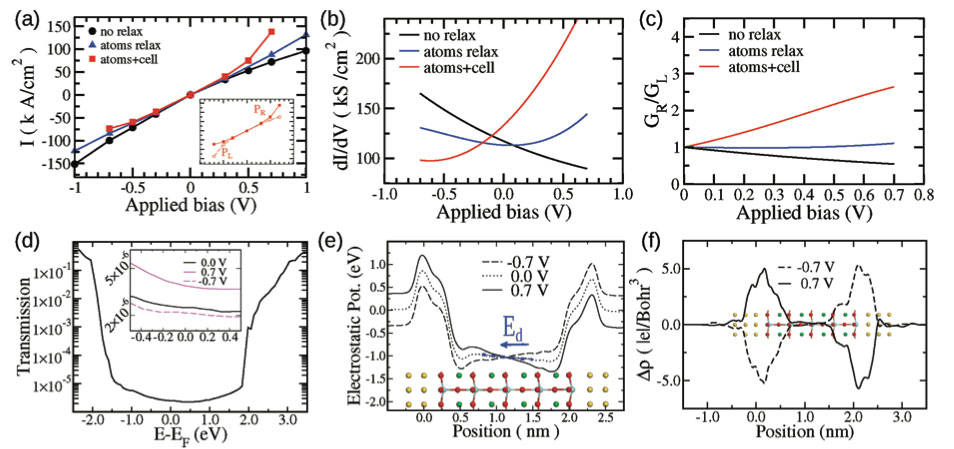### Atomistic approach addresses the Electroresistance Effect in Ferroelectric Tunnel Junctions with Symmetric Electrodes

Bilc D. I., Novaes F. D., Iñiguez J., Ordejón P. and Ghosez P., ACS Nano, 6, 1473–1478. (2012)

#### Motivation of the modeling

Understanding the effects that govern electronic transport in ferroelectric tunnel junctions (FTJs) is of vital importance to improve the efficiency of devices such as ferroelectric memories with nondestructive readout. This atomistic approach allows to quantify the contribution of different microscopic mechanisms to the electro-resistance, revealing the dominant role of the inverse piezoelectric response of the ferroelectric.

#### Achievements of the model

This first-principles study gives compelling evidence that, contrary to the common belief, it is possible to achieve a relatively large tunneling electroresistance in FTJs with symmetric electrodes. It is shown that the asymmetry of the current is essentially controlled by the piezoelectric response of the junction to an applied bias.

#### Model system/Software

The zero-bias equilibrium structure of the model s-FTJ was determined using the LDA as implemented in the code SIESTA. The electronic transport calculations in finite applied bias were performed using the non-equilibrium Green’s function formalism combined with DFT as implemented in TranSIESTA code.

The following figure compiles the main results obtained in this study:Figure: (a) IV curves corresponding to state R of the model s-FTJ and computed in various conditions. (b) Curves of dI/dV obtained by fitting the I(V) data of panel (a). (c) Ratio of the differential conductances (G = dI/dV) of the R and L states of the s-FTJ (d) Transmission function at zero bias. The inset shows the region around E-EF = 0, which determines the calculated intensities for the considered voltage values. (e) Position-dependent electrostatic potential for several voltage values (no relax case). (f) Position-dependent change of the electronic density ΔF with respect to zero-bias result (no relax case).Figure adapted from Bilc, D. I., Novaes, F. D., Iñiguez, J. , Ordejón, P. and Ghosez, P. ACS Nano, 6, 1473–1478. (2012).

Pablo Ordejón, part of the SIMUNE´s board of experts and member of the core development team of the SIESTA code is one of the authors of this work.